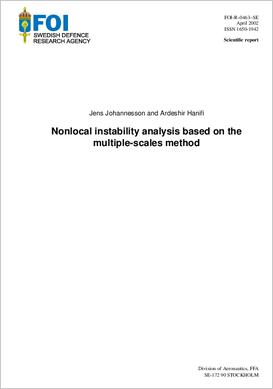# Nonlocal instability analysis based on the multiple-scales methodAuthors:

Publish date: 2002-01-01

Report number: FOI-R--0463--SE

Pages: 49

Written in: English

## Abstract

Multiple-scales technique (MSC) is used to examine the instability of non-parallel, compressible, quasi three-dimensional boundary layer flows. It models the kinematics and convective amplification of waves with weakly divergent or curved wave-rays and wave-fronts, propagating in a weakly non-uniform flow. The stability equations are put in a system of ordinary differential equations in a general orthogonal curvilinear coordinate system. The zeroth- order equations are homogeneous and govern the disturbance motion in a parallel flow and the non-local effects are calculated from the inhomogeneous first-order equations. The equations rewritten as a system of first order differential equations are discretized using compact finite difference scheme. For validation of the multiple-scales technique, we have compared the growth rates with results from ´parabolized stability equations´ (PSE).

Share page on social media